# NCERT Solutions class 12 Chemistry Solutions Part 2## myCBSEguide App

CBSE, NCERT, JEE Main, NEET-UG, NDA, Exam Papers, Question Bank, NCERT Solutions, Exemplars, Revision Notes, Free Videos, MCQ Tests & more.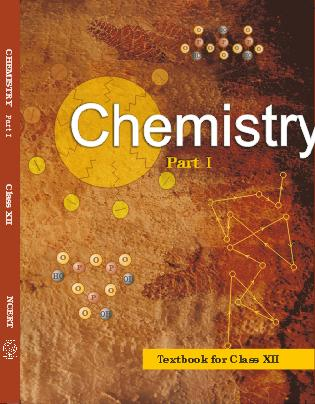## NCERT Class 12 Chemistry Chapter-wise Solutions

•  1 – The Solid State
• 2 – Solutions
• 3 – Electrochemistry
• 4 – Chemical Kinetics
• 5 – Surface Chemistry
• 6 – General Principles and Processes of Isolation of Elements
• 7 – The p-Block Elements
• 8 – The d-and f-Blocks Elements
• 9 – Coordination Compounds
• 10 – Haloalkanes and Haloarenes
• 11 – Alcohols, Phenols and Ethers
• 12 – Aldehydes, Ketones and Carboxylic Acids
• 13 – Amines
• 14 – Biomolecules
• 15 – Polymers
• 16 – Chemistry in Everyday Life

### CHAPTER TWO SOLUTIONS

• 2.1 Types of Solutions
• 2.2 Expressing Concentration of Solutions
• 2.3 Solubility
• 2.4 Vapour Pressure of Liquid Solutions
• 2.5 Ideal and Non-Ideal Solutions
• 2.6 Colligative Properties and Determination of Molar Mass
• 2.7 Abnormal Molar Masses

## NCERT Solutions class 12 Chemistry Solutions Part 2

21. Two elements A and B form compounds having formula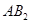and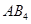. When dissolved in 20 g of benzene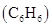, 1 g of AB2 lowers the freezing point by 2.3 K whereas 1.0 g oflowers it by 1.3 K. The molar depression constant for benzene is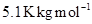. Calculate atomic masses of A and B.

22. At 300 K, 36 g of glucose present in a litre of its solution has an osmotic pressure of 4.98 bar. If the osmotic pressure of the solution is 1.52 bars at the same temperature, what would be its concentration?

23. Suggest the most important type of intermolecular attractive interaction in the following pairs.

(i) n-hexane and n-octane

(ii)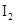and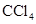(iii)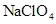and water

(iv) methanol and acetone

(v) acetonitrile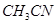and acetone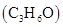.

24. Based on solute-solvent interactions, arrange the following in order of increasing solubility in n-octane and explain. Cyclohexane, KCl,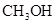,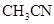.

25. Amongst the following compounds, identify which are insoluble, partially soluble and highly soluble in water?

(i) phenol

(ii) toluene

(iii) formic acid

(iv) ethylene glycol

(v) chloroform

(vi) pentanol.

26. If the density of some lake water is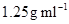and contains 92 g of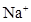ions per kg of water, calculate the molality ofions in the lake.

27. If the solubility product of CuS is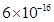, calculate the maximum molarity of CuS in aqueous solution.

28. Calculate the mass percentage of aspirin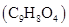in acetonitrile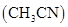when 6.5 g of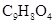is dissolved in 450 g of29. Nalorphene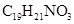, similar to morphine, is used to combat withdrawal symptoms in narcotic users. Dose of nalorphene generally given is 1.5 mg. Calculate the mass of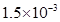m aqueous solution required for the above dose.

30. Calculate the amount of benzoic acid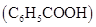required for preparing 250 mL of 0.15 M solution in methanol.

31. The depression in freezing point of water observed for the same amount of acetic acid, trichloroacetic acid and trifluoroacetic acid increases in the order given above. Explain briefly.

32. Calculate the depression in the freezing point of water when 10 g of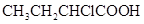is added to 250 g of water.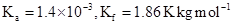.

33. 19.5 g of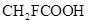is dissolved in 500 g of water. The depression in the freezing point of water observed is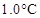. Calculate the van’t Hoff factor and dissociation constant of fluoroacetic acid.

34. Vapour pressure of water at 293 Kis 17.535 mm Hg. Calculate the vapour pressure of water at 293 K When 25 g of glucose is dissolved in 450 g of water.

35. Henry’s law constant for the molality of methane in benzene at 298 K is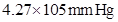. Calculate the solubility of methane in benzene at 298 K under 760 mm Hg.

36. 100 g of liquid A (molar mass 140 g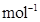) was dissolved in 1000 g of liquid B (molar mass 180 g). The vapour pressure of pure liquid B was found to be 500 torr. Calculate the vapour pressure of pure liquid A and its vapour pressure in the solution if the total vapour pressure of the solution is 475 Torr.

37. Vapor pressure of pure acetone and chloroform at 328 K are 741.8 mm Hg and 632.8 mm Hg respectively. Assuming that they form ideal solution over the entire range of composition, plot p total’ p chloroform’ and p acetone as a function of x acetone. The experimental data observed for different compositions of mixture is.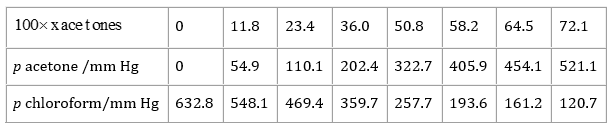38. Benzene and toluene form ideal solution over the entire range of composition. The vapour pressure of pure benzene and toluene at 300 K are 50.71 mm Hg and 32.06 mm Hg respectively. Calculate the mole fraction of benzene in vapour phase if 80 g of benzene is mixed with 100 g of toluene.

39. The air is a mixture of a number of gases. The major components are oxygen and nitrogen with approximate proportion of 20% is to 79% by volume at 298 K. The water is in equilibrium with air at a pressure of 10 atm. At 298 K if the Henry’s law constants for oxygen and nitrogen are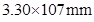and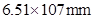respectively, calculate the composition of these gases in water.

40. Determine the amount of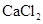(i = 2.47) dissolved in 2.5 litre of water such that its osmotic pressure is 0.75 atm at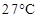.

41. Determine the osmotic pressure of a solution prepared by dissolving 25 mg of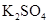in 2 liter of water at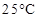, assuming that it is completely dissociated.

## NCERT Solutions class 12 Chemistry

NCERT Solutions Class 12 Chemistry PDF (Download) Free from myCBSEguide app and myCBSEguide website. Ncert solution class 12 Chemistry includes text book solutions from both part 1 and part 2. NCERT Solutions for CBSE Class 12 Chemistry have total 16 chapters. 12 Chemistry NCERT Solutions in PDF for free Download on our website. Ncert Chemistry class 12 solutions PDF and Chemistry ncert class 12 PDF solutions with latest modifications and as per the latest CBSE syllabus are only available in myCBSEguide

To download NCERT Solutions for class 12 Chemistry, Physics, Biology, History, Political Science, Economics, Geography, Computer Science, Home Science, Accountancy, Business Studies and Home Science; do check myCBSEguide app or website. myCBSEguide provides sample papers with solution, test papers for chapter-wise practice, NCERT solutions, NCERT Exemplar solutions, quick revision notes for ready reference, CBSE guess papers and CBSE important question papers. Sample Paper all are made available through the best app for CBSE students and myCBSEguide website.### 1 thought on “NCERT Solutions class 12 Chemistry Solutions Part 2”

1. it is not good